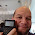## Friday, September 30, 2011

### Common Keyboard Commands for Hewlett Packard RPL Calculators (HP 48S/48G/50g)

(updated 10/1/2011)

This is a quick reference to common mathematical functions for the Hewlett Packard RPL calculators. In general, HP RPL calculators are classified into three families:

This table will focus on the HP 48S, 48G, and 50g - three of four models I actually own (I have a 49g+ which should be the same key mapping as the 50g. The 49G has a different mapping - and I do not own a 49G.)

Note: [LS] = left shift key (3rd key up from the ON button on the left side)
[RS] = right shift key (2nd key up from the ON button on the right side)

On the top row, there are six soft keys. The functions of these six keys change depending on what menu is currently active. The top row of the HP 49G, 49g+, and 50g are labeled as:

[F1] [F2] [F3] [F4] [F5] [F6]

On the HP 48S and 48G, these keys are not labeled - but I will still use the F# convention. So [F1] means first soft key from the left, [F2] means second soft key from the left, and so on.

Note: For the 50g, it is assumed that Soft Menus are turned on. (Flag -117 is set)

List of Functions

x^2
HP 48S/48G: [LS] [ √x ]
HP 50g: [LS] [ √X ]

x√y
HP 48S/48G: [RS] [√x]
HP 50g [RS] [√X]

10^x
HP 48S/48G: [LS] [y^x]
HP 50g: [LS] [EEX]

LOG
HP 48S/48G: [RS] [y^x]
HP 50g: [RS] [EEX]

e^x
HP 48S/48G: [LS] [1/x]
HP 50g: [LS] [Y^X]

LN
HP 48S/48G: [RS] [1/x]
HP 50g: [RS] [Y^X]

ABS
HP 48S: [MTH] [ F1 ] (PARTS) [ F1 ] (ABS)
HP 48G: [MTH] [F5] (REAL) [NXT] [F1] (ABS)
HP 50g: [LS] [ ÷ ]

ARG
HP 48S: [MTH] [ F1 ] (PARTS) [ F4 ] (ARG)
HP 48G: [MTH] [NXT] [F3] (CMPL) [F6] (ARG)
HP 50g: [RS] [ ÷ ]

ASIN
HP 48S/48G: [LS] [SIN]
HP 50g: [LS] [SIN]

ACOS
HP 48S/48G: [LS] [COS]
HP 50g: [LS] [COS]

ATAN
HP 48S/48G: [LS] [TAN]
HP 50g: [LS] [TAN]

->NUM
HP 48S: [RS] [EVAL]
HP 48G: [LS] [EVAL]
HP 50g: [RS] [ENTER]

HP 48S: [LS] [EVAL]
HP 48G: [LS] [ 9 ] (SYMBOLIC) [NXT] [F3]
HP 50g: [LS] [ 6 ] (CONVERT) [F4] (REWRI) [NXT] [F5] (->Q)

x!
HP 48S: [MTH] [F2] (PROB) [F3] (!)
HP 48G: [MTH] [NXT] [F1] (PROB) [F3] (!)
HP 50g: [LS] [SYMB] (MTH) [NXT] [F1] (PROB) [F3] (!)

COMB (Combination)
HP 48S: [MTH] [F2] (PROB) [F1] (COMB)
HP 48G: [MTH] [NXT] [F1] (PROB) [F1] (COMB)
HP 50g: [LS] [SYMB] (MTH) [NXT] [F1] (PROB) [F1] (COMB)

PERM (Permutation)
HP 48S: [MTH] [F2] (PROB) [F2] (PERM)
HP 48G: [MTH] [NXT] [F1] (PROB) [F2] (PERM)
HP 50g: [LS] [SYMB] (MTH) [NXT] [F1] (PROB) [F2] (PERM)

RAND (Random #)
HP 48S: [MTH] [F2] (PROB) [F4] (RAND)
HP 48G: [MTH] [NXT] [F1] (PROB) [F4] (RAND)
HP 50g: [LS] [SYMB] (MTH) [NXT] [F1] (PROB) [F4] (RAND)

% (Returns level 2 * level 1% on level 1)
HP 48S: [MTH] [F1] (PARTS) [NXT] [F4] (%)
HP 48G: [MTH] [F5] (REAL) [F1] (%)
HP 50g: [LS] [SYMB] (MTH) [F5] (REAL) [F1] (%)

%CHG (Percent Change from level 2 to level 1)
HP 48S: [MTH] [F1] (PARTS) [NXT] [F5] (%CH)
HP 48G: [MTH] [F5] (REAL) [F2] (%CH)
HP 50g: [LS] [SYMB] (MTH) [F5] (REAL) [F2] (%CH)

IP (Integer Part)
HP 48S: [MTH] [F1] (PARTS) [NXT] [NXT] [F3] (IP)
HP 48G: [MTH] [F5] (REAL) [NXT] [F5] (IP)
HP 50g: [LS] [SYMB] (MTH) [F5] (REAL) [NXT] [F5] (IP)

FP (Fraction Part)
HP 48S: [MTH] [F1] (PARTS) [NXT] [NXT] [F4] (FP)
HP 48G: [MTH] [F5] (REAL) [NXT] [F6] (FP)
HP 50g: [LS] [SYMB] (MTH) [F5] (REAL) [NXT] [F6] (FP)

To access the hyperbolic functions (SINH, COSH, etc..)
HP 48S: [MTH] [F3] (HYP)
HP 48G: [MTH] [F4] (HYP)
HP 50g: [LS] [SYMB] (MTH) [F4] (HYP)

Matrix Functions:

INV (Inverse)
HP 48S/48G: [1/x]
HP 50g: [1/X]

DET (Determinant)
HP 48S: [MTH] [F4] (MATR) [F5] (DET)
HP 48G: [MTH] [F2] (MATR) [F2] (NORM) [NXT] [F2] (DET)
HP 50g: [LS] [ 5 ] (MATRICES) [F2] (OPER) [F6] (DET)

M^T (Transpose)
HP 48S: [MTH] [F4] (MATR) [F3] (TRN)
HP 48G: [MTH] [F2] (MATR) [F1] (MAKE) [F3] (TRN)
HP 50g: [LS] [ 5 ] (MATRICES) [F2] (OPER) [NXT] [NXT] [F5] (TRN)

EGVL (Eigenvalues)
(not on the HP 48S)
HP 48G: [MTH] [F2] (MATR) [NXT] [F3] (EGVL)
HP 50g: [LS] [ 5 ] (MATRICES) [NXT] [F1] (EIGEN) [F3] (EGVL)

RREF
(not on the HP 48S)
HP 48G: [MTH] [F2] (MATR) [F3] (FACTR) [F1] (RREF)
HP 50g: [LS] [ 5 ] (MATRICES) [F5] (LIN S) [F4] (RREF)

Stack Functions:

Clear the Entire Stack
HP 48S: [RS] [backspace]
HP 48G: [LS] [DEL]
HP 50g: [RS] [Backspace]

Swap contents of levels 1 and 2
HP 48S: [LS] [right arrow] (SWAP)
HP 48G: [LS] [right arrow] (SWAP)
HP 50g: [LS] [right arrow] (unmarked)

Roll the entire stack down 1 level
(Move everything down one level and level 1 goes to stack n)
HP 48S: [PRG] [F1] (STK) [F6] (DEPTH) [F4] (ROLLD)
HP 48G: [LS] [up arrow] (STACK) [F6] (DEPTH) [F4] (ROLLD)
HP 50g: [LS] [EVAL] (PRG) [F1] [NXT] [F6] (DEPTH) [F2] (ROLLD)

Angle Conversions:

HP 48S: [LS] [SPC] (π) [ x ] 180 [ ÷ ]
HP 48G: [MTH] [F5] (REAL) [NXT] [NXT] [F5] (D->R)
HP 50g: [LS] [SYMB] (MTH) [F5] (REAL] [NXT] [NXT] [F5] (D->R)

HP 48S: 180 [ x ] [LS] [SPC] (π) [ ÷ ]
HP 48G: [MTH] [F5] (REAL) [NXT] [NXT] [F6] (R->D)
HP 50g: [LS] [SYMB] (MTH) [F5] (REAL] [NXT] [NXT] [F5] (R->D)

1.Another great tutorial! Thanks. However you left out one step to get to M^T(Transpose) in the HP50g...(LS)(5)(F2)(NXT)(NXT)(F5)

2.Thank you so much for pointing this out! I think everything should be corrected now.

Eddie

### Calculator Python: Lambda Functions

Calculator Python: Lambda Functions Introduction to Lambda Functions Lambda functions are a quick, one expression, one line, python function...